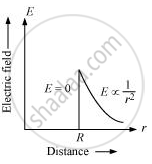Department of Pre-University Education, KarnatakaPUC Karnataka Science Class 12
Share

# Draw a graph of electric field E(r) with distance r from the centre of the shell for 0 ≤ r ≤ ∞. - Physics

#### Question

Draw a graph of electric field E(r) with distance r from the centre of the shell for 0 ≤ r ≤ ∞.

#### Solution

The graph of electric field E(r) with distance r from the centre of the shell for 0 ≤ r ≤ ∞.Is there an error in this question or solution?